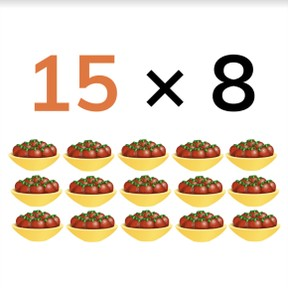Multiplying with one number to 100

# Multiplying with one number to 100

Students learn to multiply with one number to 100.

No account needed.8,000 schools use Gynzy92,000 teachers use Gynzy1,600,000 students use Gynzy

## General

The students learn to solve multiplication problems with one number to 100.

## Standards

CCSS.Math.Content.4.NBT.B.5

## Learning objective

Students will be able to multiply with one number to 100.

## Introduction

You practice the times table problems by throwing a ball to a student and stating a times table problem. The student that catches the ball gives the answer and throws the ball back.

## Instruction

First explain that a multiplication problem is a math problem in which you calculate that you have something a certain number of times. As an example you use a story in which on a property with 37 sheds there are 6 planks of wood in each shed. After this you explain how a multiplication problem is set up with the multiplication symbol, and that the first number tells how many times you have something and the second number tells how many you have each time. Next you show an example problem for which students calculate a multiplication problem with one number to 100 and can choose for themselves if they want to first multiply with the ones number or the tens number. Then you practice a few problems together with a visual aid.
Next show an abstract problem. For this one, explain that you can also first multiply the tens number or the ones number. Then you discuss how students can solve a problem and have the students practice this on their own.
Then you discuss how students can solve a story problem by following the steps in which they first see what is being asked, then find the numbers they need, then state the problem and finally solve it. Practice this together with the students as well.

Check whether the students can solve multiplication problems with one number to 100 with the questions:
- Can you flip the numbers in a multiplication problem?
- What do you calculate first when solving the problem 8 × 75?

## Quiz

The students practice their understanding of multiplication with one number to 100 through ten exercises. Some are abstract problems, some have a visual aid and some are story problems.

## Closing

Together with the students you practice another problem with a visual aid, an abstract problem and a story problem.

## Teaching tips

When students have difficulty with the learning objective, you can first have them practice with multiplication problems in which one number is between 10 and 20. In this way the problem is a bit smaller. If that is still difficult then you can have the students make groups of a concrete material to visualize the problem. After that you can make the numbers of the multiplication problem larger.

## Instruction materials

A ball

### The online teaching platform for interactive whiteboards and displays in schools

• Save time building lessons

• Manage the classroom more efficiently

• Increase student engagement